### Home > PC > Chapter 12 > Lesson 12.1.1 > Problem12-9

12-9.
1. Let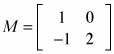and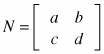. Homework Help ✎

1. Find MN.

2. Find values for a, b, c, and d so that MN = I.

3. Use the results of (b) to find M−1.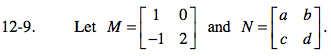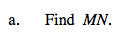$\begin{bmatrix}1(a)+0(c)&1(b)+0(d)\\-1(a)+2(c)&-1(b)+2(d)\end{bmatrix}$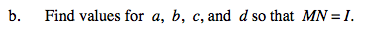$I=\begin{bmatrix}1&0\\0&1\end{bmatrix}$

Using the lower left entry from part (a) and matrix I:
−1(a) + 2(c) = 0
Now repeat this process for the rest of the entries.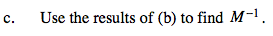Look at part (b) and remember that M(M−1) = I.